The program distributes the applied bearing load radially and non-uniformly along the cross-section of a cylindrical face or an edge of a shell.

 Sinusoidal Variation The figure shows the cross-section of a cylindrical face or a shell edge depending on your selection. For detailed illustrations of how the half-space is defined, see Bearing Loads.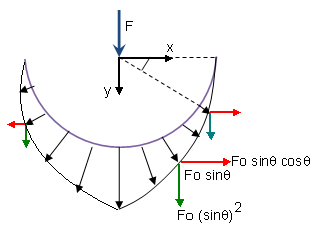You apply the load shown as F. The program transfers the load to every node along the circumference of the selected edges or cross-section of the selected faces as Fo sinΘ. The figure shows the x and y component of the transferred load by red and green arrows, respectively. The value of Fo is determined by the force equilibrium.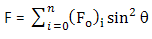n is the number of nodes along the circumference.
 Parabolic Distribution For parabolic distribution, the load is transferred to every node along the circumference as Fo (1-x2), which reduces to Fo sin2Θ.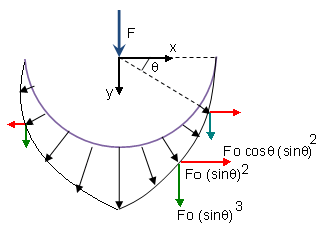The value of Fo is based on the force equilibrium requirement.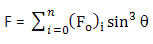Here, n is the number of nodes along the circumference. In the examples above, the horizontal component of the forces shown by red arrows balance out due to symmetrical selection of entities. It is best practice to make sure that the mesh is also symmetric.

Additional Force Applied for Unsymmetric Entities

An additional force is applied when you select unsymmetric faces or edges about the specified bearing force direction. A message alerts you when the additional forced is more than 5% of the applied bearing force.

In the example below, the selected entity is not symmetric about the specified bearing load direction. The horizontal components of the transferred load P along the circumference clearly do not sum to zero.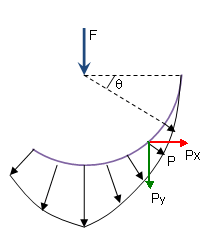The additional unbalanced load is calculated by the following equation: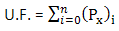U.F. denotes the unbalanced force.

For sinusoidal load distribution:

Px = P cosΘ = Fo sinΘ cosΘ

For parabolic load distribution:

Px = P cosΘ = Fo sin2Θ cosΘ

The percentage of unbalanced load is calculated as (U.F./F) x 100.

In general, the mesh refinement and the location of the node affect the calculation of the unbalanced force due to the variation of Px along the circumference.